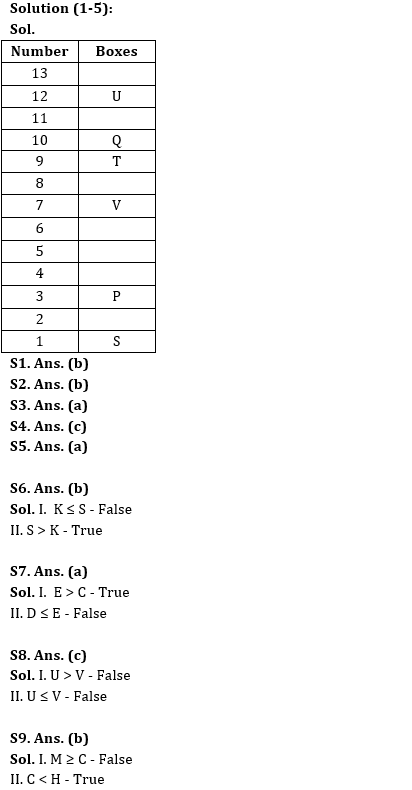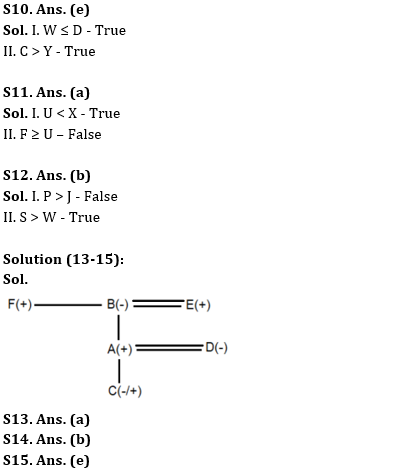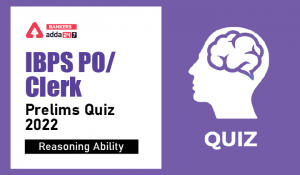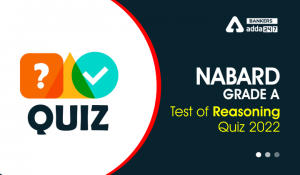Latest Banking jobs   »

# Reasoning Ability Quiz For IBPS RRB PO Clerk Prelims 2022- 15th July

Directions (1-5): Answer the questions based on the information given below.
A certain number of boxes are stacked one above another such that the bottommost box is numbered as 1 and the box above it is 2 and so on. V is placed 4th to the above of P which is a prime numbered box. One box is placed between P and S. Number of boxes placed above V is one more than the number of boxes between V and S. T is placed 2nd to the above of V. U is placed 2nd from the top. Number of boxes between U and T is equal to the number of boxes below P.

Reasoning Quiz For IBPS RRB PO Clerk Prelims 2022 15th July IN HINDI

Q1. How many boxes are there in the stack?
(a) 11
(b) 13
(c) 10
(d) 12
(e) None of these

Q2. How many boxes are placed below P?
(a) 1
(b) 2
(c) 4
(d) 6
(e) None of these

Q3. Which of the following box is the 7th numbered box?
(a) V
(b) T
(c) S
(d) Either V or T
(e) None of the above

Q4. If a box Q is placed just above T, then how many boxes are placed between Q and U?
(a) Two
(b) Four
(c) One
(d) Three
(e) None of the above

Q5. How many boxes are placed between S and T?
(a) 7
(b) 5
(c) 3
(d) 4
(e) None of the above

Directions (6-8): In the following questions assuming the given statement to be true, find which of the conclusion(s) among given conclusions is/are definitely true and then give your answers accordingly.

Q6. Statements: L > N ≥ K< P, O > S, S > R ≥ L
Conclusions:
I. K ≤ S
II. S > K
(a) Only I is true
(b) Only II is true
(c) Either I or II is true
(d) Neither I nor II is true
(e) Both I and II are true

Q7. Statements: A > B ≥ M< D, E> Q, Q > C ≥ D
Conclusions:
I. E > C
II. D ≤ E
(a) Only I is true
(b) Only II is true
(c) Either I or II is true
(d) Neither I nor II is true
(e) Both I and II are true

Q8. Statements: S > U ≥ M< P, O > Q, Q > V ≥ P
Conclusions:
I. U > V
II. U ≤ V
(a) Only I is true
(b) Only II is true
(c) Either I or II is true
(d) Neither I nor II is true
(e) Both I and II are true

Directions (9-12): In the following questions assuming the given statement to be true, find which of the conclusion(s) among given conclusions is/are definitely true and then give your answers accordingly.

Q9. Statements: M ≥ N = H; N > J ≥ C
Conclusions:
I. M ≥ C
II. C < H
(a) Only I is true
(b) Only II is true
(c) Either I or II is true
(d) Neither I nor II is true
(e) Both I and II are true

Q10. Statements: Y < D = C ≥ S ≥ W > U = V
Conclusions:
I. W ≤ D
II. C > Y
(a) Only I is true
(b) Only II is true
(c) Either I or II is true
(d) Neither I nor II is true
(e) Both I and II are true

Q11. Statements: Z ≤ C < X; Z ≥ U; X ≤ F
Conclusions:
I. U < X
II. F ≥ U
(a) Only I is true
(b) Only II is true
(c) Either I or II is true
(d) Neither I nor II is true
(e) Both I and II are true

Q12. Statements: P > W ≤ U = J, T < S ≥ P < J
Conclusions:
I. P > J
II. S > W
(a) Only I is true
(b) Only II is true
(c) Either I or II is true
(d) Neither I nor II is true
(e) Both I and II are true

Directions (13-15): Answer the questions based on the information given below.
There are six members A, B, C, D, E and F in the family of three generations. There is no single parent in the family. B is mother-in-law of D. A is father of C. F is brother-in-law of E. F is the maternal uncle of A.

Q13. Who is the husband of B?
(a) E
(b) A
(c) D
(d) C
(e) F

Q14. How is D related to C?
(a) Sister
(b) Mother
(c) Father
(d) Brother
(e) Sister-in-law

Q15. How many females member are there in the family?
(a) Two
(b) Three
(c) Four
(d) More than four
(e) Can’t be determined

Solutions#### Congratulations!Incorrect details? Fill the form again here

•Reasoning Ability Quiz For IBPS Clerk/PO...
•Reasoning Ability Quiz For IBPS RRB PO C...
•Reasoning Ability Quiz For NABARD Grade ...
•Reasoning Ability Quiz For IBPS Clerk Pr...
•Reasoning Ability Quiz For IBPS Clerk Pr...
•Reasoning Ability Quiz For NABARD Grade ...